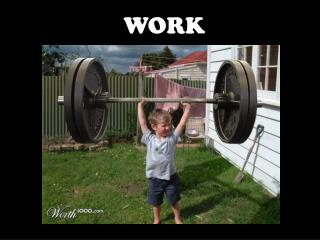DownloadDownload PresentationWORK

# WORK

Télécharger la présentation## WORK

- - - - - - - - - - - - - - - - - - - - - - - - - - - E N D - - - - - - - - - - - - - - - - - - - - - - - - - - -
##### Presentation Transcript

1. WORK

2. What is it? Shoveling the walkway is hard work! Writing this report is hard work! What do you do for work? Becoming good at the violin takes a lot of work.

3. Work is done when a force causes an object to move in the direction of the force. In science, WORK has a very specific meaning:

4. 2 things determine if work is being done: • A FORCE is applied to the object. • The object MOVESin the direction of the force.

5. Workor Not Work?

6. ☐Pushing one of Mr. Dingcrates’ crates across the floor. Work or not work??? ☐Lifting a barbell off the ground. ☐Holding the barbell stationary over your head.

7. The Math… The formula for work: Work = Force x Distance The distance over which the force is applied (in meters) The force applied to the object (in Newtons) • The unit of work is a joule (rhymes with “cool”) • 1 joule (J) is the work done pushing an object a distance of 1 meter with a force of 1 Newton.

8. Example

9. You try it! • How much work is done if you push your little brother on his bicycle for 10 meters with a force of 50 Newtons? • How much work do you do on a bowling ball that weighs 80 N if you lift it 1.5 meters off the ground? • How much work do you do on that same bowling ball if you carry it 20 meters horizontally across the room?

10. Question: If the force of the gun powder on the cannon ball is the same for both cannons: Is the work done on each cannon ball the same? Will one cannon ball go farther? Newton’s Laws are only the beginning… 2m long 4m long Hint: The force of the gunpowder pushes the cannon ball until it leaves the barrel of the cannon.

11. Force applied for a distance of 2 meters Work = force x 2m 2m long The longer cannon does 2x the work on the cannon ball since the force is applied over 2x the distance! 4m long Force applied for a distance of 4 meters Work = force x 4m

12. Newton tells us: The force of the exploding gunpowder accelerates the ball. (a=f/m) The concept of work tells us: The forceacting over a longer distance results in more work done on the ball. So, Why is this important????

13. ….Work is the transfer of energy to an object • So…since the longer cannon did 2x the work on the cannon ball, the cannon ball will have gained 2x the energy,and will: • leave the cannon barrel with a greater velocity • do more damage when it hits its target

14. Work Quick-Lab Choose a few objects. Measure their mass in kg and convert it to weight in N. Then lift the object a certain distance and calculate the work you did on the object. To convert Kg to Newtons: 1kg = 9.8 Newtons